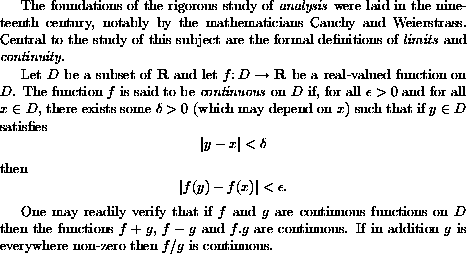A Typical LaTeX Input File

The LaTeX program reads in text from a suitably prepared input file, and creates a DVI file' which encodes information on the fonts to be used and the positioning of the characters on the printed page. There are many programs available that can translate the DVI file' into page description languages such as PostScript', or convert it into the format appropriate for previewing the document on a computer screen or printing it out on dot-matrix printers.

Here is an example of a typical LaTeX input file:

\documentclass[a4paper,12pt]{article}
\begin{document}

The foundations of the rigorous study of \emph{analysis}
were laid in the nineteenth century, notably by the
mathematicians Cauchy and Weierstrass. Central to the
study of this subject are the formal definitions of
\emph{limits} and \emph{continuity}.

Let $D$ be a subset of $\bf R$ and let
$f \colon D \to \mathbf{R}$ be a real-valued function on
$D$. The function $f$ is said to be \emph{continuous} on
$D$ if, for all $\epsilon > 0$ and for all $x \in D$,
there exists some $\delta > 0$ (which may depend on $x$)
such that if $y \in D$ satisfies
$|y - x| < \delta$
then
$|f(y) - f(x)| < \epsilon.$

One may readily verify that if $f$ and $g$ are continuous
functions on $D$ then the functions $f+g$, $f-g$ and
$f.g$ are continuous. If in addition $g$ is everywhere
non-zero then $f/g$ is continuous.

\end{document}

When we apply LaTeX to these paragraphs we produce the textThis example illustrates various features of LaTeX. Note that the lines

\documentclass[a4paper,12pt]{article}
\begin{document}
are placed at the beginning of the input file. These are followed by the main body of the text, followed by the concluding line
\end{document}
Note also that, although most characters occurring in this file have their usual meaning, there are characters such as \, \$, { and } which have special meanings within LaTeX. In particular, there are sequences of characters which begin with a backslash' \ which are used to produce mathematical symbols and Greek letters and to accomplish tasks such as changing fonts. These are known as control sequences.